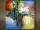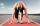# Donuts

Find how many donuts each student will receive if you share 126 donuts in a ratio of 1:5:8

Result

a =  9
b =  45
c =  72

#### Solution:Leave us a comment of example and its solution (i.e. if it is still somewhat unclear...):

Showing 0 comments:Be the first to comment!## Next similar examples:

1. Two numbersFind two numbers whose difference and ratio is 2.
2. AgesJohn, Teresa, Daniel and Paul have summary 56 years. Their ages are in a ratio of 1:2:5:6. Determine how many years have each of them.
3. Line segmentThe 4 cm long line segment is enlarged in the ratio of 5/2. How many centimeters will measure the new line segment?
4. Arble bagA marble bag sold by Rachel's Marble Company contains 5 orange marbles for every 6 green marbles. If a bag has 35 orange marbles, how many green marbles does it contain?
5. Apples 2James has 13 apples. He has 30 percent more apples than Sam. How many apples has Sam?
6. Sales offGoods is worth € 70 and the price of goods fell two weeks in a row by 10%. How many % decreased overall?
7. PeppersIn the box are yellow (a), green (b) and red (c) peppers. Their amount is in a ratio 2:4:1 . Most are yellow peppers and green the least. Calculate the number of peppers each type if the total number of peppers is 70.
8. RunnersFor three runners (on the first to third place) is prepared 30 chocolate that they be distributed in the ratio of 3 : 2 : 1 How much chocolate will get everyone?
9. New refrigeratorNew refrigerator sells for 1024 USD, Monday will be 25% discount. How much USD will save, and what will be the price?
10. Three workersThree workers got paid for work. First earn € 50, the second € 100 and third € 60. For further work should receive together € 735. How do you divide that amount in order to be distributed in the same proportion as the first job?
11. Image scaleThe actual image dimensions are 60 cm x 80cm and has a reduced size 3 cm x 4 cm. At what scale the image was reduced?
12. Land areaA land area of Asia and Africa are in a 3: 2 ratio, the European and African are is 1:3. What are the proportions of Asia, Africa, and Europe?
13. 1.5 divided1.5 divided by 1 = w divided by 4
14. Runner 2Marian run 12 meters in 8 seconds. How far would Marian run for 70 seconds, if still run at the same pace?
15. Ratio v2Decrease in the ratio 12:16 number 13.2.
16. Masons1 mason casts 30.8 meters square in 8 hours. How long casts 4 masons 178 meters square?
17. GlovesI have a box with two hundred pieces of gloves in total, split into ten parcels of twenty pieces, and I sell three parcels. What percent of the total amount I sold?